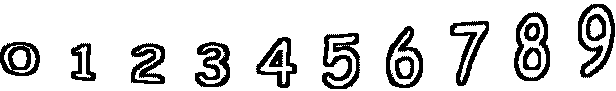5 - 0 =        6 - 0 =9 - 6 =        1 - 0 =        5 - 1 =        7 - 3 = 5 - 1 =        3 - 3 =        4 - 0 =        2 - 0 =